[email protected] +1 203-349-9909 / +91-8080042523

# How to use Roundup Function in Excel

Roundup Function in Excel can use as a variety of formulas to round numbers in Excel depending on the situation. We have ROUND, ROUNDUP, ROUNDDOWN, MROUND, INT, TRUNC, CEILING, FLOOR, FIXED, EVEN, ODD and a few more. Here, we will learn how to Round up numbers in Excel.

Before we start, we must know about the Roundup function.

## What is Round UP Function?

A function that rounds a number up, away from 0 (zero). The ROUNDUP function in excel is a built-in function in Excel that rounds numbers up to a given number of decimal places. It can be used as a worksheet function in Excel. As a worksheet function, the ROUNDUP function can be entered as part of a formula in a cell of a worksheet.

## Why Use Round Up?

To Round a number up to a given number of digits.

Syntax:

=ROUNDUP (number, num_digits)

number – The number to round up.

num_digits – The number of digits to which number should be rounded up.

Return value: A rounded number.

Now let’s learn how to use Roundup function in Excel in the following steps: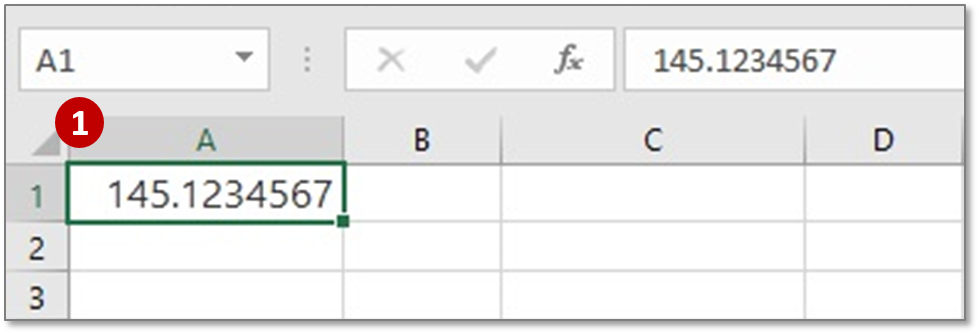Step 1: Open an excel sheet and type any random value, like 145.1234567 in a cell.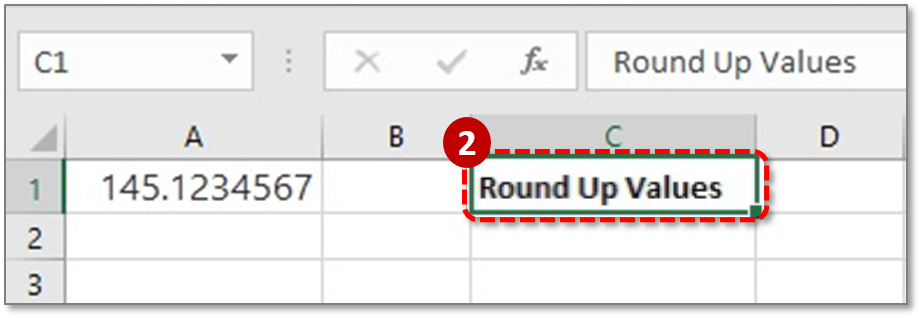Step 2: In cell C1 type a heading of this column Round Up Values. We will use our Round up formulas under this column.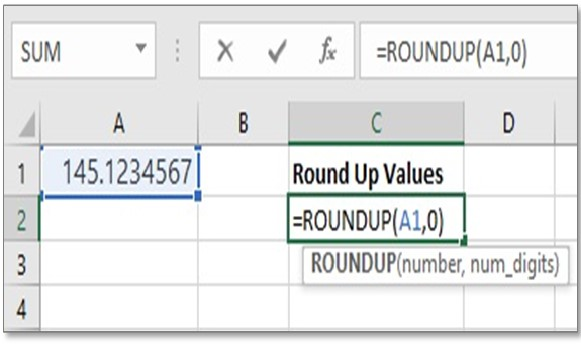Step 3: Type a Round Up formula in cell C2: =ROUNDUP(A1,0)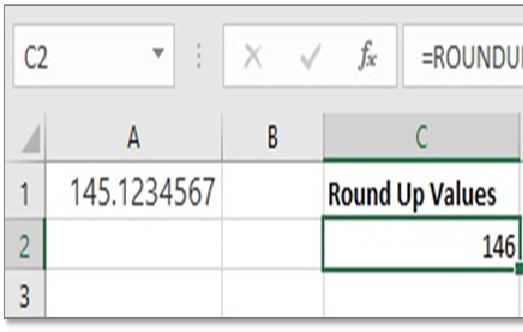Step 4: Now press ENTER. Cell C2 will show the round up the value of 145.1234567 as 146.

=ROUNDUP(A1,0) Rounds 145.1234567 (The value of cell A1) up to zero decimal places for which the value returned as 146 in cell C2.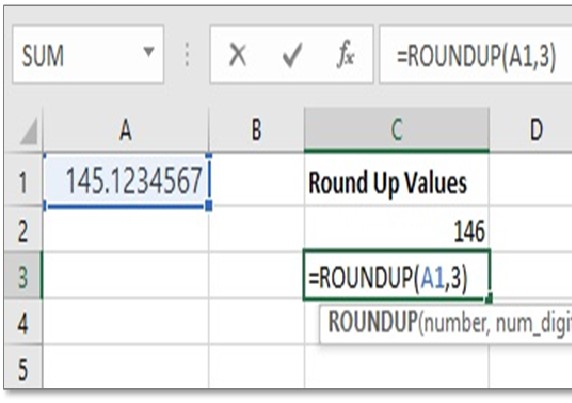Step 5: Now type another Round Up formula in cell C3:

=ROUNDUP(A1,3)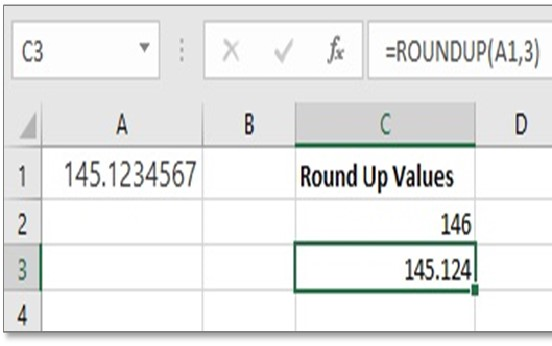Step 6: Press ENTER. Now the rounded up value is showing 145.124.

=ROUNDUP(A1, 3) Rounds the value 145.1234567 up to three decimal places and result returned as 145.124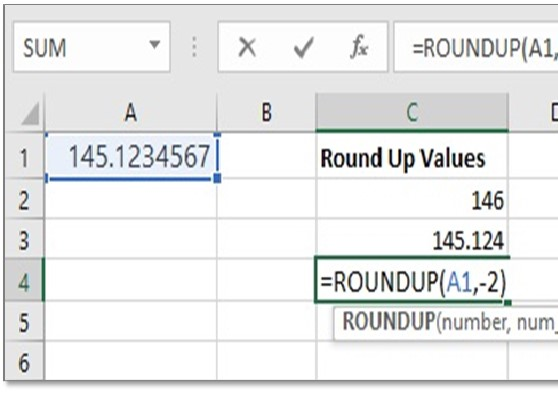Step 7: Now type another Round Up formula in cell C4:

=ROUNDUP(A1,-2)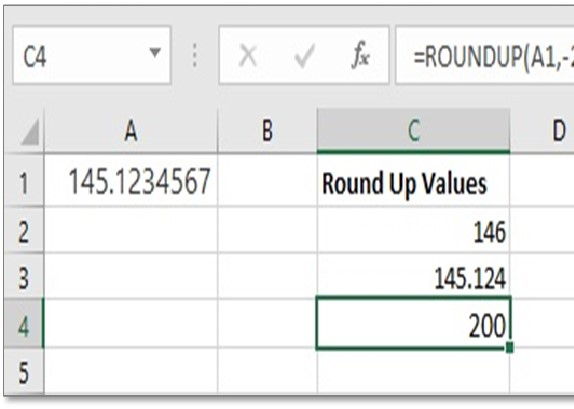Step 8: Press ENTER. Now the round up value is showing 200.

Formula =ROUNDUP(A1, -2) Rounds 1451234567 up to 2 decimal places to the left of the decimal point and result returned as 200.

Tip 1: Remember,

If num_digits > 0, number is rounded up to the specified number of decimal places to the right of the decimal point.

If num_digits < 0, number is rounded up to the left of the decimal point (i.e. to the nearest 10, 100, 1000, etc.).

If num_digits = 0, number is rounded up to the nearest integer.

Tip 2: There are some another Round Example you can use also if need:

Example 1: Round to nearest million with one decimal point

(312789123 to 312.8)

=ROUND(A1/1000000,1)

This formula divides the number by million (1,000,000) and then round this to 1 decimal point.

Example 2: Get next even number

(42.1 to 44)

=EVEN(A1)

Gets you the next EVEN number (away from zero) 44.

Example 3: Get next odd number

(44.93 to 45)

=ODD(A1)

Gets you the next ODD number (away from zero) 45.

Example 4: Round to next 9 (i.e 19,29,39 etc.)

(23 to 29)

=ROUNDUP(A1,-1)-1

To do this, we just round up the number to next 10 and then subtract 1 from it.

Hope you liked it. Comment your take on the ROUNDUP Function in Excel.